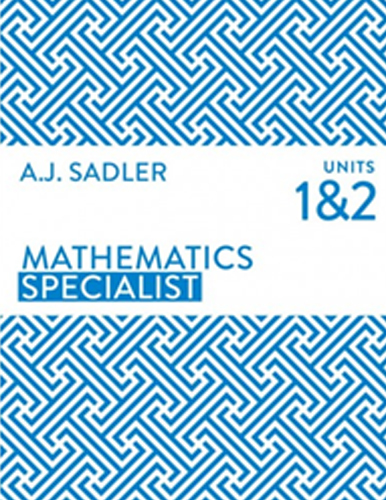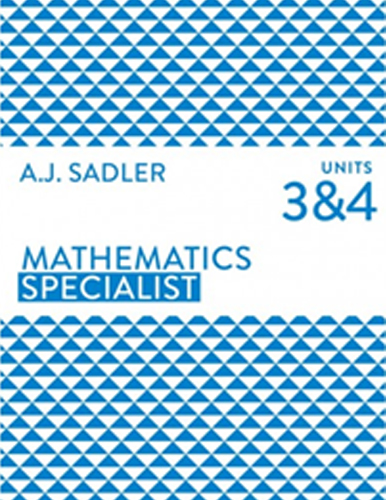## Mathematics Specialist

Home / Mathematics Specialist

# Mathematics Specialist

## Mathematics Specialist (ATAR) Tutor Perth

At Tutor Perth we hand pick the finest Maths Specialist home tutors in Perth. Our Maths Specialist tutors are engaging role models, mentors and are genuine specialists at teaching maths. We teach in accordance to the WA curriculum framework and provide comprehensive tutoring solutions to the A.J Sadler suite of text books, units 1, 2, 3 and 4.

Our engaging maths tutors help students achieve top ATAR results in the following A.J Sadler units:

## Year 11 Mathematics Specialist (ATAR) | Specialist Tutors

### We Tutor – Units 1 & 2

• True or false Statements
• Counting
• Vectors
• Vectors in component form
• Geometric proofs
• Relative displacement and relative velocity
• Proofs using vectors
• Scalar product
• Trigonometrical identities and equations
• Matrices
• Transformation matrices
• Proofs
• Complex numbers## Year 12 Mathematics Specialist (ATAR) | Specialist Tutors### We Tutor – Units 3 & 4

• Complex numbers
• Polar form of a complex number
• Functions
• Vector equation of a line in the x-y plane
• Vectors in three dimensions
• Systems of linear equations
• Vector calculus
• Differentiation techniques and applications
• Integration techniques and applications
• Differential equations
• Simple harmonic motion
• Sample means